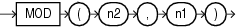# MOD

SyntaxDescription of the illustration mod.gif

Purpose

`MOD` returns the remainder of `n2` divided by `n1`. Returns `n2` if `n1` is 0.

This function takes as arguments any numeric datatype or any nonnumeric datatype that can be implicitly converted to a numeric datatype. Oracle determines the argument with the highest numeric precedence, implicitly converts the remaining arguments to that datatype, and returns that datatype.

Table 2-10, "Implicit Type Conversion Matrix" for more information on implicit conversion and "Numeric Precedence" for information on numeric precedence

Examples

The following example returns the remainder of 11 divided by 4:

```SELECT MOD(11,4) "Modulus" FROM DUAL;

Modulus
----------
3
```

This function behaves differently from the classical mathematical modulus function when `m` is negative. The classical modulus can be expressed using the `MOD` function with this formula:

```m - n * FLOOR(m/n)
```

The following table illustrates the difference between the `MOD` function and the classical modulus:

m n MOD(m,n) Classical Modulus
`11` `4` `3` `3`
`11` `-4` `3` `-1`
`-11` `4` `-3` `1`
`-11` `-4` `-3` `-3`

FLOOR and REMAINDER, which is similar to `MOD`, but uses `ROUND` in its formula instead of `FLOOR`